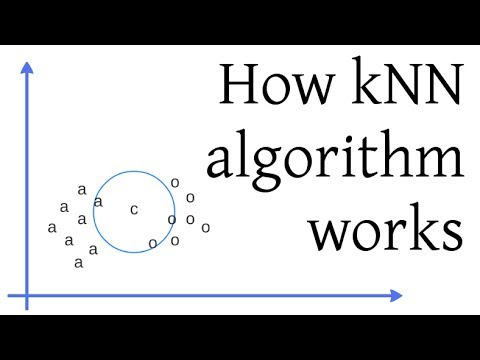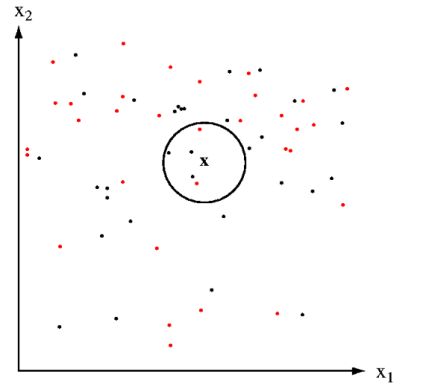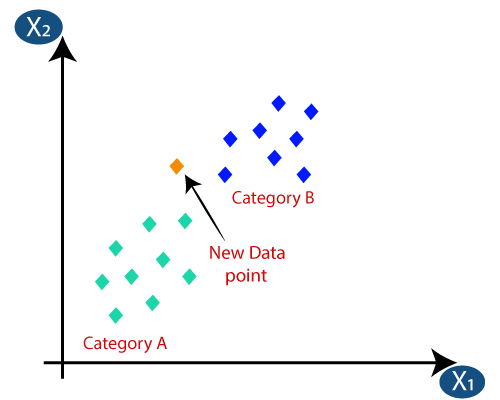1610971200

# How KNN Algorithm Works

In this video I describe how the k Nearest Neighbors algorithm works, and provide a simple example using 2-dimensional data and k = 3.

#knn #deep-learning #machine-learning

## Buddha Community1593436560

## KNN Algorithm: What?When?Why?How?

KNN: K Nearest Neighbor is one of the fundamental algorithms in machine learning. Machine learning models use a set of input values to predict output values. KNN is one of the simplest forms of machine learning algorithms mostly used for classification. It classifies the data point on how its neighbor is classified.

KNN classifies the new data points based on the similarity measure of the earlier stored data points. For example, if we have a dataset of tomatoes and bananas. KNN will store similar measures like shape and color. When a new object comes it will check its similarity with the color (red or yellow) and shape.

K in KNN represents the number of the nearest neighbors we used to classify new data points.

How do I choose K?

In real-life problems where we have many points the question arises is how to select the value of K?

Choosing the right value of K is called parameter tuning and it’s necessary for better results. By choosing the value of K we square root the total number of data points available in the dataset.

a. K = sqrt (total number of data points).

b. Odd value of K is always selected to avoid confusion between 2 classes.

When is KNN?

a. We have properly labeled data. For example, if we are predicting someone is having diabetes or not the final label can be 1 or 0. It cannot be NaN or -1.

b. Data is noise-free. For the diabetes data set we cannot have a Glucose level as 0 or 10000. It’s practically impossible.

c. Small dataset.

How does KNN work?

We usually use Euclidean distance to calculate the nearest neighbor. If we have two points (x, y) and (a, b). The formula for Euclidean distance (d) will be

d = sqrt((x-a)²+(y-b)²)

We try to get the smallest Euclidean distance and based on the number of smaller distances we perform our calculation.

Let’s try KNN on one database to see how it works. The data can be extracted from https://github.com/adityakumar529/Coursera_Capstone/blob/master/diabetes.csv.

``````import pandas as pd
import numpy as np
from sklearn.model_selection import train_test_split
from sklearn.preprocessing import StandardScaler
from sklearn.neighbors import KNeighborsClassifier
from sklearn.metrics import confusion_matrix
from sklearn.metrics import accuracy_score
from sklearn.metrics import f1_score
``````

#knn-algorithm #data-science #knn #towards-data-science #machine-learning #algorithms1594957980

# What is k-Nearest Neighbor?

It is one of the simplest supervise learning techniques. If there is a new data point that we need to classify then we will choose k “closest” data points (nearest neighbors) that will be around the new data point for performing classification or regression. K can be any positive number.

KNN is a Non-parametric algorithm i.e. it does not make any underlying assumptions about the distribution of data. It is used for both classifications and regression problems.Example: We have two categorical variables red and black, and we have to classify a new data point X whether it belongs to red or black.

We select K=5 and select five close data points near to X. As you can see in the above figure in 5 data point 3 is black and 2 is red since black in a majority in number the new data point X will belong to a black class.

**KNN for Regression: **When KNN is used for regression problems the prediction is based on the mean or the median of the nearest neighbor.

**KNN for Classification: **Inclassification, the output can be calculated as the class with the highest occurrence from the K nearest neighbor.

# How does the KNN algorithm works?

The KNN algorithm work with the below steps:

• Step-1: Select the number K of the neighbors
• Step-2: Calculate the distance of K number of neighbors
• Step-3: Select the K nearest neighbors as per the calculated distance.
• Step-4: Among these k neighbors, count the number of the data points in each category.
• Step-5: Assign the new data points to that category for which have the highest number.

Suppose we have a new data point and we need to put the new data point in the correct category. Consider the below image:• First, we need to choose the K number of neighbors, so we will choose the k=5.
• Next, we will calculate the distance between the data points.

We can any of the three functions to calculate the distance between the data point to find the closest data point. In this example, we will use the Euclidean distance.

#knn #data-science #knn-algorithm #algorithms1614329473

## Fix: G Suite not Working | G Suite Email not Working | Google Business

G Suite is one of the Google products, developed form of Google Apps. It is a single platform to hold cloud computing, collaboration tools, productivity, software, and products. While using it, many a time, it’s not working, and users have a question– How to fix G Suite not working on iPhone? It can be resolved easily by restarting the device, and if unable to do so, you can reach our specialists whenever you want.
For more details: https://contactforhelp.com/blog/how-to-fix-the-g-suite-email-not-working-issue/

#g suite email not working #g suite email not working on iphone #g suite email not working on android #suite email not working on windows 10 #g suite email not working on mac #g suite email not syncing

1607930471

## Xfinity Stream Not Working?

Xfinity, the tradename of Comcast Cable Communications, LLC, is the first rate supplier of Internet, satellite TV, phone, and remote administrations in the United States. Presented in 2010, previously these administrations were given under the Comcast brand umbrella. Xfinity makes a universe of mind boggling amusement and innovation benefits that joins a great many individuals to the encounters and minutes that issue them the most. Since Xfinity is the greatest supplier of link administrations and home Internet in the United States, it isn’t amazing that the organization gets a ton of investigating and inquiry goal demands on its telephone based Xfinity Customer Service.

#my internet is not working comcast #comcast tv remote not working #my xfinity internet is not working #xfinity stream not working #xfinity wifi hotspot not working1624970880

## Reptile: OpenAI’s Latest Meta-Learning Algorithm

As more data, better algorithms, and higher computing power continue to shape the future of artificial intelligence (AI), reliable machine learning models have become paramount to optimise outcomes. OpenAI’s meta-learning algorithm, Reptile, is one such model designed to perform a wide array of tasks.

For those unaware, meta-learning refers to the idea of ‘learning to learn by solving multiple tasks, like how humans learn. Using meta-learning, you can design models that can learn new skills or adapt to new environments rapidly with a few training examples.

In the recent past, the meta-learning algorithm has had a fair bit of success as it can learn with limited quantities of data. Unlike other learning models like reinforcement learning, which uses reward mechanisms for each action, meta-learning can generalise to different scenarios by separating a specified task into two functions.

The first function often gives a quick response within a specific task, while the second function includes the extraction of information learned from previous tasks. It is similar to how humans behave, where they often gain knowledge from previous unrelated tasks or experiences.

Typically, there are three common approaches to meta-learning.

1. Metric-based: Learn an efficient distance metric
2. Model-based: Use (recurrent) network with external or internal memory
3. Optimisation-based: Optimise the model parameters explicitly for fast learning

For instance, the above image depicts the model-agnostic meta-learning algorithm (MAML) developed by researchers at the University of California, Berkeley, in partnership with OpenAI. The MAML optimises for a representation θ that can quickly adapt to new tasks.

On the other hand, Reptile utilises a stochastic gradient descent (SGD) to initialise the model’s parameters instead of performing several computations that are often resource-consuming. In other words, it also reduces the dependency of higher computational hardware requirements, if implemented in a machine learning project.

#developers corner #how reptile works #meta learning algorithm #meta-learning algorithm #algorithm